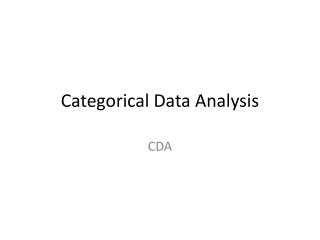DownloadDownload PresentationCategorical Data Analysis

# Categorical Data Analysis

Télécharger la présentation## Categorical Data Analysis

- - - - - - - - - - - - - - - - - - - - - - - - - - - E N D - - - - - - - - - - - - - - - - - - - - - - - - - - -
##### Presentation Transcript

1. Outline • Contingency Table • Graphical display of Categorical Data Bar Chart, Pie Chart, Mosaic Plot • Measures of Association Pearson Correlation Coefficient, Cramer’s V • Test of Independence • Test of Symmetry

2. Contingency Table • A contingency table is a rectangular table having I rows for categories of X and J columns for categories of Y. • The cells of the table represent the I×J possible outcomes.

3. Contingency Table: Example 1_Heart attack vs. Aspirin use • The table below is from a report on the relationship between aspirin use and heart attacks by the Physicians’ Health Study Research Group at Harvard Medical School. • The 2×3 contingency table is

4. Generating Contingency Table in R • Input the 2×3 table in R as a 2×3 matrix • Change the matrix to table using the function as.table(), because some functions are happier with tables than matrices

5. Graphical Display of Categorical Data • One Categorical Variable Bar Chart: a chart with rectangular bars with lengths proportional to the values that they represent Pie Chart: a circular chart divided into sectors, illustrating proportion.

6. Graphical Display of Categorical Data • Two Categorical Variables Mosaic Plot: a graphical display that examine the relationship among two or more categorical variables.

7. Mosaic Plot Construction • A mosaic plot starts with a square with length one. The square is divided firstly into horizontal bars whose widths are proportional to the probabilities associated with the first categorical variable. Then each bar is split vertically into bars that are proportional to the conditional probabilities of the second categorical variables. Additional splits can be made if wanted using a third, fourth variable, etc.

8. Mosaic Plot: Example 2_HairEyeColor • The HiarEyeColor data comes from a survey of students at the University of Delaware (1974). It has 592 observations on 3 variables (Hair, Eye, Sex). Here we omit Sex.

9. Mosaic Plot in R • Option 1: install package vcd, use function mosaic() • Option 2: use function mosaicplot()

10. Measures of Association • Continuous Variables-Pearson Correlation Coefficient • Ordinal Variables-Pearson Correlation Coefficient • Nominal Variables-Cramer’s V

11. Cramer’s V • Cramer’s V measures the association between two nominal variables. It varies from 0 (no association) to 1 (complete association) and can reach 1 only when the two variables are equal to each other.

12. Cramer’s V (cont’d) Comments: 1, When the two variables are binary, Cramer’s V is the same as Phi Coefficient (which measures the association between two binary variables) 2, In R, under library(vcd), use function assocstats()

13. Contingency Table Analysis • Large Sample Size Chi-square Test • Small Sample Size Fisher’s Exact Test

14. Test of Independence (Chi-square Test) H0: Row and Column are independent πij=πi+π+j for all i,j Ha: Row and Column are not independent πij≠πi+π+j for some i and j

15. Test of Independence (Chi-square Test) Under H0: πij=πi+π+j for all i,j Expected Counts in each cell is

16. Test of Independence (Fisher’s Exact Test) • When any of the expected counts fall below 5, Chi-square test is not appropriate. Instead, we use Fisher’s Exact Test. Example 3: The following data are from a Stanford University study of the effectiveness of the antidepressant Celexa in the treatment of compulsive shopping.

17. Test of Independence in R • Chi-Square Test Use R function chisq.test() • Fisher’s Exact Test Use R function fisher.test()

18. Test of Symmetry: Matched Pairs • Example 4: Suppose two surveys on President’s job approval were conducted one-month apart on 1600 Americans and the result is summarized in the following table. (Source: Agresti, 1990) Is there a significant difference in job approval rating?

19. Test of Symmetry: Matched Pairs

20. Useful Resource • Quick R http://www.statmethods.net/index.html